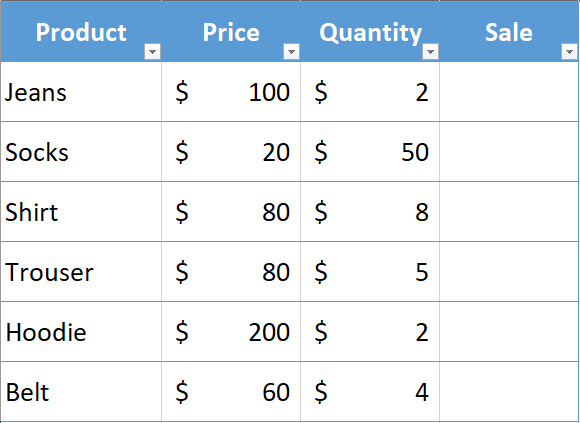# How to Multiply One Column by Another in Excel

Excel offers several functions that enable you to perform both simple and complex arithmetic operations. One of the most common operations in Excel is multiplying. This can be accomplished in several different ways. In this tutorial, we will see several quick and simple methods for multiplying two columns in Excel.

To better understand the concept, let’s look at a sample dataset.Here we will multiply the Price column by the Quantity column to get our sales values for each product.

## Method 1 – Multiply Using Simple Arithmethic Multiplication

The easiest way to multiply two columns in an Excel sheet is using an asterisk (*) symbol. Follow the steps below to multiply your columns.

## Step 1 – Place Equals To Sign (=)

• Select the cell where you want to calculate the sales amount & place equals to sign.

## Step 2 – Type The Formula

• To multiply columns in cell D2, type the following formula.

=B2*C2.

• Press enter.

## Step 3 – Drag The Formula Down Below

• To find out sales of all products drag the formula to the remaining cells.

## Method 2 – Multiply Two Columns Using Product Function

Another quick method to multiply two columns in excel is to use the Product Function. You can follow the below steps to find out the sales. The PRODUCT function multiplies all the numbers given as arguments and returns the product.

Syntax & Argument

PRODUCT(number1, [number2], …)

Number 1: Required. The first number or range that you want to multiply.

Number 2: Required. Optional. Additional numbers or ranges that you want to multiply.

## Step 1 – Place Equals To Sign (=)

• Select the cell where you want to calculate the sales amount & place equals to sign.

## Step 2 – Start Typing The Formula

• For this example, we will use the following formula.

=Product(B2:C2)

• Type the above formula in cell D2. During the use of the PRODUCT function, we can select desired cells using a colon (:) or comma (,).
• Press enter.

## Step 3 – Copy & Paste The Formula Downwards

• Copy & paste the formula down the cells to calculate the sales.

## Method 3 – Multiply Two Columns With an Array Formula

Another way to multiply two columns in excel is the Array formula. It’s a simple and easy method.

First, we will select the Price column then place an asterisk sign (*) and then select the Quantity column.

=B2:B7*C2:C7

## Step 1 – Select all the cells and write the formula in the first cell

• Select all the cells where we want to implement the formula.
• Start typing the above formula in cell D2.

## Step 2 – Fill down The Formula In the Cells Below

• To implement the formula to all the selected cells at once, enter the formula by pressing the CTRL+SHIFT+ENTER keys together.
• The formula will be implemented in all the cells.